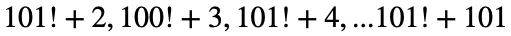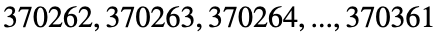# Problem 52634. Easy Sequences 14: Consecutive Composites

Generating a given number of consecutive numbers all of which are composites is easy. For example the following is a sequence of 100 consecutive composite numbers:These numbers are huge. The first number of the sequence is around 9.4259 × 10 ^ 159. A smaller solution can be found by using "primorials" instead of factorials. Primorial is the product of all primes less than or equal to a given number. If we use primorials the first number is reduced to:
>> prod(primes(101)) + 2
>> ans =
2.3286e+38
Although, this is a significant size reduction, the smallest possible 100 consecutive composites series, is still way less than that. The smallest 100 consecutive composites series,starts with 370262, that is:if we define the set 'C' as the smallest consecutive 'n' composite numbers, the function 'f(n)' as the smallest element in 'C', and 'F' is the set that contains all f(x)'s for x = 1,2,3...n, write the function S(n), which is the sum of all elements of F.

### Solution Stats

36.84% Correct | 63.16% Incorrect
Last Solution submitted on Nov 16, 2023

### Community Treasure Hunt

Find the treasures in MATLAB Central and discover how the community can help you!

Start Hunting!